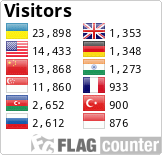DOI: https://doi.org/10.24028/gzh.0203-3100.v38i4.2016.107811

Modified additive-averaged splitting for solving three-dimensional equations of hydrodynamics

L. N. Katsalova

Abstract

Hydrodynamic equations form the basis of modern ecological and meteorological models. The complexity of the implementation of such models is due to three-dimensionality and nonlinearity of the equations, as well as large amounts of data and the need for prompt solutions. The use of parallel computing for solving hydrodynamic systems entered in the world practice. This approach makes it possible to reduce solution time significantly, but requires the development of new methods of implementation of the model equations. The described method for solving three-dimensional equations of convective diffusion is a modification of additive-averaged splitting three-dimensional equations. The modification carried out to increase the efficiency of splitting for the parallel computing. The essence of the modification is the introducing a parameter that indicates the number of steps, on which one-dimensional problems are solved by an explicit account in parallel on different processors without exchange of data between them. The results of numerical experiments that confirm the good accuracy, convergence and efficiency of the proposed method are shown.

Keywords

hydrodynamics; convection diffusion equation; parallel computing; additive-averaged splitting; explicit account method

PDF (Русский)

References

Belov P. N., Borisenkov E. P., Panin B. D., 1989. Numerical methods of weather forecasting. Leningrad: Gidrometeoizdat, 376 p. (in Russian).

Gill A., 1986. The dynamics of the atmosphere and ocean. Moscow: Mir, 416 p. (in Russian).

Gladkiy A. V., Skopetskiy V. V., 2005. Methods of numerical modeling of environmental processes: Textbook. Kyiv: NTU "KPI", 148 p. (in Ukrainian).

Gordeziani D. G., Meladze G. V., 1974. Simulation of the third boundary value problem for mul¬ti¬di¬men¬si-o¬nal parabolic equations in an arbitrary domain by one-dimensional equations. Zhurnal vychislitelnoy matematiki i matematicheskoy fiziki (1), 246—250 (in Russian).

Huk L. M. 2011. Explicit account method for rea¬li¬za¬tion of atmospheric circulation model. Visnyk Kyivskogo natsionalnogo universiteta im. Tarasa Shevchenka. Ser. fiz.-mat. nauky (4), 102—106 (in Ukrainian).

Doroshenko A. Yu., 2000. Mathematical models and methods of high-performance parallel computing. Algebrodynamical approach. Kiev: Naukova Dumka, 177 p. (in Russian).

Katsalova L. N., 2015. The study of convergence of additive-averaged splitting based on the scheme of explicit solution for three-dimensional equations of convective diffusion. Geofizicheskiy zhurnal 37(6), 131—136 (in Ukrainian).

Katsalova L. N., 2013. One method of implementation of simplified atmospheric circulation model. Visnyk Kyivskogo natsionalnogo universiteta im. Tarasa Shevchenka. Ser. fiz.-mat. nauky (1), 178—171 (in Ukrainian).

Kibel I. A., 1957. Introduction to hydrodynamic methods of short-term weather forecasting. Moscow: Gostekhizdat, 375 p. (in Russian).

Marchuk G. I., 1967. Numerical methods in weather forecasting. Leningrad: Gidrometeoizdat. 353 p. (in Russian).

Prusov V. A., Doroshenko A. Yu., 2006. Modelling of natural and anthropogenic processes in the atmosphere. Kyiv: Naukova Dumka, 542 p. (in Ukrainian).

Prusov V. A., Doroshenko A. Yu., Chernysh R. I., 2009a. Selecting of parameter of modified additive-averaged method. Kibernetika i sistemnyy analiz (4), 98—105 (in Russian).

Prusov V. A., Doroshenko A. Yu., Chernysh R. I., 2009b. The method of numerical solution of multidimensional problem of convective diffusion. Kibernetika i sistemnyy analiz (1), 100—107 (in Russian).

Prusov V. A., Doroshenko A. Yu., Chernysh R. I., Guk L. N., 2007. Efficient difference scheme numerical solution of the convective diffusion problem. Kibernetika i sistemnyy analiz (3), 64—74 (in Russian).

Prusov V. A. Snizhko S. I., 2005. Mathematical modeling of atmospheric processes: Textbook. Kyiv: Nika-Tsentr, 496 p. (in Ukrainian).

Samarskiy A. A., Vabishchevich P. N., 1999. Numerical methods for solving convection-diffusion problems. Moscow: Editorial URSS, 248 p. (in Russian).

Samarskiy A. A., Gulin A. V., 1973. Stability of difference schemes. Moscow: Nauka, 416 p. (in Russian).

Samarskiy A. A., Mikhaylov A. P., 2001. Mathematical modeling: Ideas. Methods. Examples. Moscow: Fizmatlit, 320 p. (in Russian).

Chernysh R. I., 2009. Modified additive-averaged splitting scheme. Conference of Young Scientists from the modern problems of mechanics and mathematics named after Y. S. Pidstryhach, May 25—27, 2009: abstracts. Lviv, P. 242 (in Ukrainian).

Schumann W., Groetzbach G., Kleiser L., 1984. Direct methods for the numerical simulation of turbulent flows. In: Methods of calculation turbulent flows. Moscow: Mir, P. 103—226 (in Russian).

Anderson D. A., Tannehill J. C., Pletcher R. H., 1997. Computational fluid mechanics and heat transfer. Second Edition. Taylor and Francis, 792 p.

Roache P. J., 1985. Computational Fluid Dynamics. Albuquerque: Hermosa Publ., 616 p.